# Volumes and areas of body surfaces

Volumes and areas of body surfaces: prism, parallelepiped, right
prism, right-angled parallelepiped, cube, pyramid, regular pyramid,
truncated pyramid, regular truncated pyramid, circular cylinder,
round cylinder, circular cone, round cone, truncated circular cone,
truncated round cone, sphere (ball), hemisphere, spherical segment,
spherical layer, spherical sector, hollow ball.

Designations: V – a volume;   S – a base area;   S lat – a lateral surface area; P – a full surface area;   h – a height;   a, b, c – dimensions of a right angled parallelepiped;   A – an apothem of a regular pyramid and a regular truncated pyramid;   L – a generatrix of a cone;   p –  a perimeter or a circumference of a base;  r – a radius of a base;   d – a diameter of a base;  R – a radius of a ball; D – a diameter of a ball;   indices  1  and  2  are related to radii, diameters, perimeters and areas of upper and lower bases of truncated prism and pyramid.

A prism ( right and oblique ) and a parallelepiped:

V = Sh .

A right prism:

S lat = ph .

A right angled parallelepiped:

V = abc ;    P = 2 ( ab + bc + ac ) .

A cube:

V = a ³ ;    P = 6 a ² .

A pyramid ( regular and irregular ) :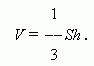A regular pyramid:A truncated pyramid ( regular and irregular ) :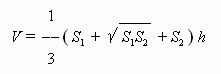A regular truncated pyramid: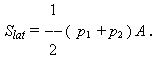A circular cylinder ( right and oblique ) :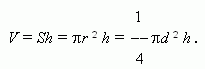A round cylinder :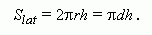A circular cone ( round and oblique) :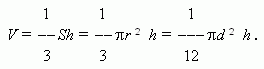A round cone: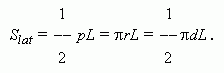A truncated circular cone ( round and oblique ) :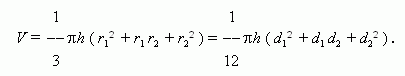A truncated round cone: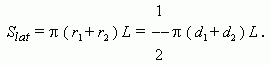A sphere ( ball ):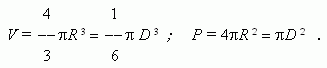A hemisphere: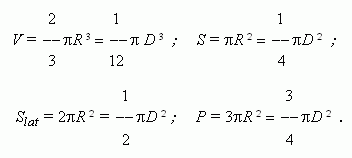A spherical segment: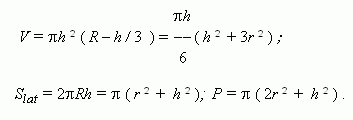A spherical layer: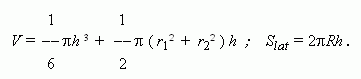A spherical sector: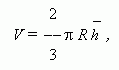_
here h  – a height of a segment, contained in the sector .

A hollow ball: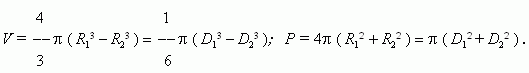here R 1 , R 2 , D 1 , D 2 radii and diameters of external and internal spherical surfaces correspondingly.